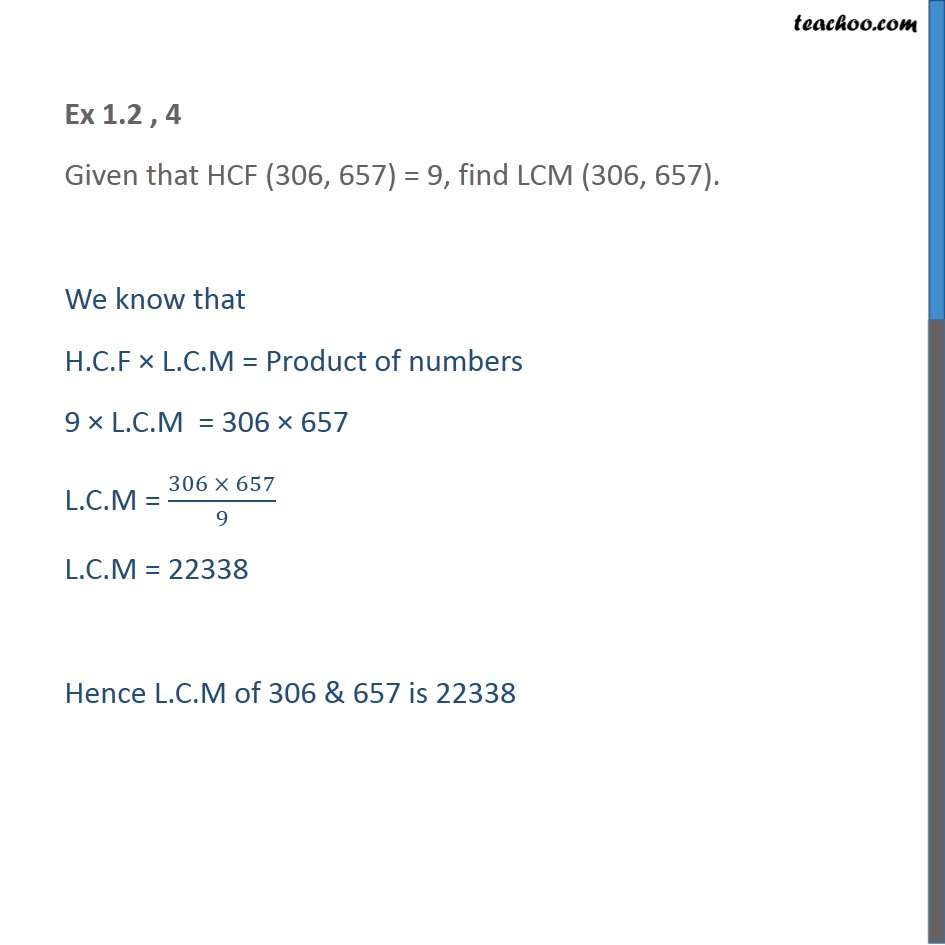1. Class 10
2. Important Questions for Exam - Class 10
3. Chapter 1 Class 10 Real Numbers

Transcript

Ex 1.2 , 4 Given that HCF (306, 657) = 9, find LCM (306, 657). We know that H.C.F × L.C.M = Product of numbers 9 × L.C.M = 306 × 657 L.C.M = (306 × 657)/9 L.C.M = 22338 Hence L.C.M of 306 & 657 is 22338

Chapter 1 Class 10 Real Numbers

Class 10
Important Questions for Exam - Class 10